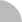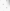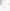# 臺灣博碩士論文加值系統

(44.192.94.86) 您好！臺灣時間：2022/07/03 20:34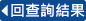:::

### 詳目顯示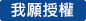: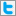Twitter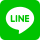•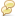被引用:0
•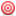點閱:125
•評分: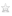•下載:0
•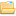書目收藏:0
 給定 λ 為單位閉圓裡的複數，H是可分離的希爾伯特空間，且 ε={e_n:n=0,1,2,…} 為H一單範正交基底。在H裡，一有界算子T若滿足< Te_{m+1},Te_{n+1} >=λ< Te_m,Te_n > ( <•,•> 為H裡的內積 )，則稱T為 λ-托普立茲算子。這主題源自於最近研究一個特例算子方程式S*AS = λA + B, 這裡的S是在H裡的shift, 對於在l^2(Z)裡找一有界矩陣 (a_{ij})滿足以下方程式扮演著不可或缺的角色a_{2i,2j} = p_{ij} + aa_{ij}a_{2i,2j−1} = q_{ij} + ba_{ij}a_{2i−1,2j} = v_{ij} + ca_{ij}a_{2i−1,2j−1} = w_{ij} + da_{ij}所有 i, j ∈ Z, (p_{ij}), (q_{ij}), (v_{ij}), (w_{ij}) 皆為在 l^2(Z) 裡的有界矩陣且a, b, c, d ∈C。當S是一unilateral shift，我們可以精確的得到所熟知的托普立茲算子為S*AS = A的解。這篇文章主要是討論 λ-托普立茲算子的一些基本性質，像是有界性和緊致性，並且比較相關結果與托普立茲算子差異。
 Let λ be a complex number in the closed unit disc, and H be a separable Hilbert space with the orthonormal basis, say, ε={e_n:n=0,1,2,…}. A bounded operator T on H is called a λ-Toeplitz operator if < Te_{m+1},Te_{n+1} >=λ< Te_m,Te_n > (where <•,•> is the inner product on H).The subject arises just recently from a special case of the operator equation S*AS = λA + B, where S is a shift on H, which plays an essential role in finding bounded matrix (a_{ij}) on l^2(Z) that solves the system of equationsa_{2i,2j} = p_{ij} + aa_{ij}a_{2i,2j−1} = q_{ij} + ba_{ij}a_{2i−1,2j} = v_{ij} + ca_{ij}a_{2i−1,2j−1} = w_{ij} + da_{ij}for all i, j ∈ Z, where (p_{ij}), (q_{ij}), (v_{ij}), (w_{ij}) are bounded matrices on l^2(Z) and a, b, c, d ∈C.It is also clear that the well-known Toeplitz operators are precisely the solutions of S*AS = A, when S is the unilateral shift. The purpose of this paper is to discuss some basic topics, such as boundedness and compactness, of the λ-Toeplitz operators, and study the similarities and the differences with the corresponding results for the Toeplitz operators.
 1. Introduction 32. Boundedness of T_{λ,φ} 63. Compactness of T_{λ,φ} 10
  A. Brown and P. R. Halmos, Algebraic properties of Toeplitz operators, J. reine angew. Math., 231, 1963, pp.89-102. M. D. Contreras and A. G. Hern′andaz-D′ıaz, Weighted composition operators between different Hardy spaces, Integral Equations and Operator Theory, 46, 2003, pp.165-188. M. D. Contreras and A. G. Hern′andaz-D′ıaz, Weighted composition operators on space of functions with derivatives in a Hardy space, J. Operator Theory, 52, 2004, pp.173-184. C. C. Cowen and E. Ko, Weighted composition operators on H^2, Preprint. G. Gunatillake, Spectrum of a compact weighted composition operator, Proc. AMS, 135, no. 2, 2007, pp.461-467. G. Gunatillake, Compact weighted composition operators on the Hardy space, Proc. AMS, 136, no. 8, 2008, pp.2895-2899. M. C. Ho, Adjoints of slant Toeplitz operators II, Integral Equations and Operator Theory, 41, 2001, pp.179-188. M. C. Ho and M. M. Wong, Operators that commute with slant Toeplitz operators, Applied Math. Research eXpress, 2008, Article ID abn003, 20 pages, doi:10.1093/amrx/abn003. M. C. Ho and C. M. Hsu, On infinite matrices on l^2(Z) generated dyadically by a 2 × 2 block, submitted. C. M. Hsu, On infinite matrices whose entries satisfying certain dyadic recurrent formula, master thesis, NSYSU, 2007. B. D. MacCluer and R. Zhao, Essential norms of weighted composition operators between Bloch-type spaces, Rocky Mountain J. Math., 33, no. 4, 2003. S. Ohno, Weighted composition operators between H^1 and the Bloch space, Taiwanese J.Math., 5, no. 3, 2001, pp.555-563.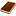國圖紙本論文推文當script無法執行時可按︰推文 網路書籤當script無法執行時可按︰網路書籤 推薦當script無法執行時可按︰推薦 評分當script無法執行時可按︰評分 引用網址當script無法執行時可按︰引用網址 轉寄當script無法執行時可按︰轉寄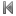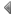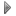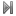top
 無相關論文

 無相關期刊

 1 λ-托普立茲算子之 Fredholm 譜 2 解析 λ-托普立茲 算子 3 托普立茲-組合算子之結構 4 某算子方程式之齊次詳解 5 有關無窮矩陣之項滿足某些二冪次遞迴公式的討論 6 哈弟不等式在加權哈弟空間上的研究 7 加權合成算子在平方可積函數空間相關於一個正測度的結構 8 取樣算子之Perron-Frobenius定理及其譜半徑公式的誤差估計 9 由移算子所引出在B(H)上的一個映射之“特徵向量” 10 對於對稱模函數所生成之函數空間之富氏分析 11 B(pi)空間及其對偶 12 對於slantToeplitz算子為常數的算子 13 支援協作擴增實境的霧結構單目定位與建圖系統 14 咖啡渣的修飾及其在抗菌性能之研究 15 金融裁罰與銀行獲利能力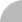簡易查詢 | 進階查詢 | 熱門排行 | 我的研究室